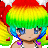Birthday: 04/18

## Contact

• Send Message

My name is Iara , but you can just call me Cookie.

I'm His Dork' heart

If I'm offline and you wanna talk to me , just send me a PM.

I'm random. I love randomness. Always did , Always will. mrgreen

## Friends

View All Friends

••••••••••Report | 06/25/2011 4:34 am`ola :):):):):):):):):):):):):):):):):):):):):):):):):):):):):):):):):):):):):):):):):):):):):):):):):):):):):):):):):):):):):):):):):):):):):):):):):):):):):):):):):):):):):):):):):):):):):):):):):):):):):):):):):):):):):):):):):):):):):):):):):):):):):):):):):):):):):):):):):):):):):):):):):):):):):):):):):):):):):):):):):):):):):):):):):):):):):):):):):):):):):):):):):):):):):):):):):):):):):):):):):):):):):):):):):):):):):):):):):):):):):):):):):):):):):):):):):):):):):):):):):):):):):):):):):):):):):):):):):):):):):):):):):):):):):):):):):):):):):):):):):):):):):):):):):):):):):):):):):):):):):):):):):):):):):):):):):):):):):):):):):):):):):):):):):):):):):):):):):):):):):):):):):):):):):):):):):):):):):):):):):):):):):):):):):):):):):):):):):):):):):):):):):):):):):):):):):):):):):):):):):):):):):):):):):):):):):):):):):):):):):):):):):):):):):):):):):):):):):):):):):):):):):):):):):):):):):):):):):):):):):):):):):):):):):):):):):):):):):):):):):):):):):):):):):):):):):):):):):):):):):):):):):):):):):):):):):):):):):):):):):):):):):):):):):):):):):):):):):):):):):):):):):):):):):):):):):):):):):):):):):):):):):):):):):):):):):):):):):):):):):):):):):):):):):):):):):):):):):):):):):):):):):):):):):):):):):):):):):):):):):):):):):):):):):):):):):):):):):):):):):):):):):):):):):):):):):):):):):):):):):):):):):):):):):):):):):):):):):):):):):):):):):):):):):):):):):):):):):):):):):):):):):):):):):):):):):):):):):):):):):):):):):):):):):):):):):):):):):):):):):):):):):):):):):):):):):):):):):):):):):):):):):):):):):):):):):):):):):):):):):):):):):):):):):):):):):):):):):):):):):):):):):):):):):):):):):):):):):):):):):):):):):):):):):):):):):):):):):):):):):):):):):):):):):):):):):):):):):):):):):):):):):):):):):):):):):):):):):):):):):):):):):):):):):):):):):):):):):):):):):):):):):):):):):):):):):):):):):):):):):):):):):):):):):):):):):):):):):):):):):):):):):):):):):):):):):):):):):):):):):):):):):):):):):):):):):):):):):):):):):):):):):):):):):):):):):):):):):):):):):):):):):):):):):):):):):):):):):):):):):):):):):):):):):):):):):):):):):):):):):):):):):):):):):):):):):):):):):):):):):):):):):):):):):):):):):):):):):):):):):):):):):):):):):):):):):):):):):):):):):):):):):):):):):):):):):):):):):):):):):):):):):):):):):):):):):):):):):):):):):):):):):):):):):):):):):):):):):):):):):):):):):):):):):):):):):):):):):):):):):):):):):):):):):):):):):):):):):):):):):):):):):):):):):):):):):):):):):):):):):):):):):):):):):):):):):):):):):):):):):):):):):):):):):):):):):):):):):):):):):):):):):):):):):):):):):):):):):):):):):):):):):):):):):):):):):):):):):):):):):):):):):):):):):):):):):):):):):):):):):):):):):):):):):):):):):):):):):):):):):):):):):):):):):):):):):):):):):):):):):):):):):):):):):):):):):):):):):):):):):):):):):):):):):):):):):):):):):):):):):):):):):):):):):):):):):):):):):):):):):):):):):):):):):):):):):):):):):):):):):):):):):):):):):):):):):):):):):):):):):):):):):):):):):):):):):):):):):):):):):):):):):):):):):):):):):):):):):):):):):):):):):):):):):):):):):):):):):):):):):):):):):):):):):):):):):):):):):):):):):):):):):):):):):):):):):):):):):):):):):):):):):):):):):):):):):):):):):):):):):):):):):):):):):):):):):):):):):):):):):):):):):):):):):):):):):):):):):):):):):):):):):):):):):):):):):):):):):):):):):):):):):):):):):):):):):):):):):):):):):):):):):):):):):):):):):):):):):):):):):):):):):):):):):):):):):):):):):):):):):):):):):):):):):):):):):):):):):):):):):):):):):):):):):):):):):):):):):):):):):):):):):):):):):):):):):):):):):):):):):):):):):):):):):):):):):):):):):):):):):):):):):):):):):):):):):):):):):):):):):):):):):):):):):):):):):):):):):):):):):):):):):):):):):):):):):):):):):):):):):):):):):):):):):):):):):):):):):):):):):):):):):):):):):):):):):):):):):):):):):):):):):):):):):):):):):):):):):):):):):):):):):):):):):):):):):):):):):):):):):):):):):):):):):):):):):):):):):):):):):):):):):):):):):):):):):):):):):):):):):):):):):):):):):):):):):):):):):):):):):):):):):):):):):):):):):):):):):):)`
Report | 06/24/2011 1:01 pmi'm changing my name to XxMiStErSwAgGaxX
Report | 04/18/2011 9:12 amhappy birthday
Report | 02/14/2011 7:25 amWeclome wink
Report | 02/14/2011 12:00 amHappy Valentines Day heart
Report | 02/13/2011 12:24 pmHEY IARA ! xp THANK GOD NO SCHOOL I HOPE MISS CASSIDY COMES BACK ON THURSDAY rolleyes WHAT YOU DOING??? 4laugh I HAVE TO GO OFLINE AT 9:00 LAWL blaugh
Report | 02/13/2011 8:24 amYEA I SAW IT YESTRDAY IT WAS AWESOME!!!!!!!!!!!!!!!!!! HAVE U SEEN IT YET?
Report | 02/12/2011 10:24 ami like ur profile
Report | 02/10/2011 1:05 amu look pretty heart

## Signature

I believe in Jesus Christ, my Savior. If you do too, copy and paste this into your signature. heart heart

heart ♥♥♥♥♥♥♥♥♥♥♥♥♥ I LOVE JUSTIN DREW BIEBER ♥♥♥♥♥♥♥♥♥♥♥♥♥ heart

## Recent VisitorsRaNdOmNeSS